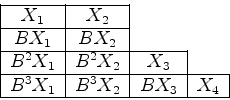### A Simple Jordan Canonical Form Algorithm

This document is intended for anyone who has been exposed to a second course in linear algebra and who has been mystified by the usual lengthy treatments of the Jordan canonical form and who simply wants an algorithm which can be implemented by an exact arithmetic matrix calculator such as my CMAT. For the moment, we omit proofs and present such an algorithm for finding a nonsingular matrix P such that P-1AP=JA, the Jordan canonical form of A. (Proofs can be found in my 1991 MP274 lecture notes.)

### Motivation

Let A be an n x n matrix over a field F and let the characteristic polynomial chA(x) split completely as a product of linear factors over F. Let c be an eigenvalue of A having algebraic multiplicity a and geometric multiplicity g. Then A is similar to a direct sum of elementary Jordan matrices; that is matrices Je(c), with c on the diagonal, 1 on the subdiagonal and 0 elsewhere.
Let B = A - cIn. Also let N(Bh) stand for the null space of Bh. Then N(B) and N(Ba) are the eigenspace and generalized eigenspace of A, corresponding to the eigenvalue c.
Let nh=dim(N(Bh)). Then g=n1 ≤ na=a. If g=a for all eigenvalues, then JA is diagonal and there are no difficulties. However if g < a, the situation is more complicated.

Our aim is to choose a basis for N(B) having the form

Be1-1X1, . . . , Beg-1Xg,           (1)

where e1≥ · · · ≥ eg≥1 and e1+ · · · +eg=a.
For then the following generalized eigenvectors:

X1, BX1, . . . , Be1-1X1;

·
·
·
Xg, BXg, . . . , Beg-1Xg

will together form a basis for the generalized eigenspace N(Ba). (See for example either the aforementioned MP274 notes or Lemma, p. 308, Linear Algebra, S.H. Friedberg, A.J. Insel, L.E. Spence, Prentice-Hall 1979.)
Then if Pc is the matrix whose columns are formed from the above list, we have

APc = Pcdiag(Je1(c), . . . , Jeg(c)).

We then let P be the result of juxtaposing the Pc for the distinct eigenvalues. Then P will be a nonsingular n x n matrix and P-1AP=JA.

It helps to visualize the generalized eigenvectors in the following tabular form:If e1,...ek are the exponents >= h, the vectors Be1-1X1, . . . , Bek-1Xk, can be shown to form a basis for Nh, the intersection of C(Bh-1) (the column space of Bh-1) and the eigenspace N(B).
In fact k = nh - nh-1.

The numbers e1, . . . , eg are called the Segre characteristic for the eigenvalue c and form a "vertical" partition of the algebraic multiplicity a. The numbers nh increase strictly and then become equal:

n1<· · · < nb = · · · = na = a.

Here b=e1.
The numbers µh = nh - nh-1 decrease monotonically:

µ1 = n1≥· · · ≥ µb

and are called the Weyr characteristic for the eigenvalue c and form a "horizontal" partition of a.

We remark that the vectors BjXi,i=1,...,g,  j=0,...,ei-1, form a basis for N(Bh).

### The construction

The above discussion shows that the subspaces Nh hold the key to the construction of the basis (1). We start with a basis for Nb and extend to bases of the successive distinct Nh, until a basis is obtained for N1 of the form (1). The process is facilitated by the observation that

Nh = < Bh-1Y1, . . . , Bh-1Yr >,

if Y1, . . . , Yr is a basis for N(Bh). We also make use of the "Left-To-Right" algorithm, which selects a basis from a list of spanning vectors.

### An example

Let chA(x)=x11. Also suppose that n1 = 4, n2 = 7, n3 = 9, n4 = 11.
Then µ1 = n1 = 4, µ2 = n2 - n1 = 3, µ3 = n3 - n2 = 2, µ4 = n4 - n3 = 2.
Also B=A and our table of generalized eigenvectors isA basis for N4:
N(B4) = <Y1, . . . , Y11> and hence N4= < B3Y1, . . . , B3Y11>.
The Left-To-Right algorithm will give: N4= < B3X1, B3X2>.
A basis for N2:
N(B2) = <Z1, . . . , Z7>, so
N2= < BZ1, . . . , BZ7> = < B3X1, B3X2, BZ1, . . . , BZ7>.
The Left-To-Right algorithm gives: N2= < B3X1, B3X2, BX3>.
A basis for N1:
N(B) = <W1, . . . , W4> = < B3X1, B3X2, BX3, W1, . . . , W4>.
The Left-To-Right algorithm gives: N(B) = < B3X1, B3X2, BX3, X4 >.
Then P = [ X1| BX1| B2X1| B3X1| X2| BX2| B2X2| B3X2| X3| BX3| X4] has the property that

P-1AP = diag(J4(0), J4(0), J2(0), J1(0)).